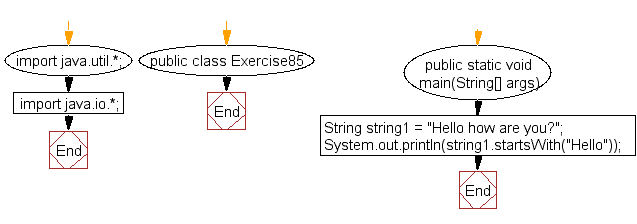﻿ Java exercises: Check if a string starts with a specified word - w3resource# Java Exercises: Check if a string starts with a specified word

## Java Basic: Exercise-85 with Solution

Write a Java program to check if a string starts with a specified word.

Sample Data: string1 = "Hello how are you?"

Pictorial Presentation:Sample Solution:

Java Code:

``````import java.util.*;
import java.io.*;
public class Exercise85 {
public static void main(String[] args)
{
String string1 = "Hello how are you?";
System.out.println(string1.startsWith("Hello"));
}
}
```
```

Sample Output:

```true
```

Flowchart:Java Code Editor:

What is the difficulty level of this exercise?

Test your Programming skills with w3resource's quiz.

﻿

## Java: Tips of the Day

countOccurrences

Counts the occurrences of a value in an array.

Use Arrays.stream().filter().count() to count total number of values that equals the specified value.

```public static long countOccurrences(int[] numbers, int value) {
return Arrays.stream(numbers)
.filter(number -> number == value)
.count();
}
```

Ref: https://bit.ly/3kCAgLb